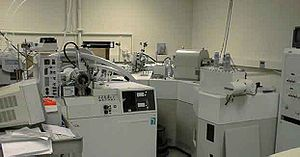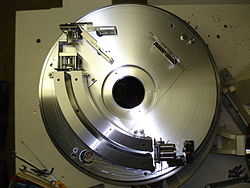# Sector instrument

﻿
Sector instrumentA five sector mass spectrometer

A sector instrument is a general term for a class of mass spectrometer that uses a static electric or magnetic sector or some combination of the two (separately in space) as a mass analyzer. A popular combination of these sectors has been the BEB (magnetic-electric-magnetic). Most modern sector instruments are double focusing instruments in that they focus the ion beams both in direction and velocity.

## Theory

The behavior of ions in a homogeneous, linear, static electric or magnetic field (separately) as is found in a sector instrument is simple. The physics are described by a single equation called the Lorentz force law. This equation is the fundamental equation of all mass spectrometric techniques and applies in non-linear, non-homogeneous cases too and is an important equation in the field of electrodynamics generally.$\mathbf{F} = q (\mathbf{E} + \mathbf{v} \times \mathbf{B}),$

where E is the electric field strength, B is the magnetic field induction, q is the charge of the particle, v is its current velocity (expressed as a vector), and × is the cross product.

So the force on an ion in a linear homogeous electric field (an electric sector) is:$F=qE\,$,

in the direction of the electric field, with positive ions and opposite that with negative ions.Electric sector from a Finnigan MAT mass spectrometer (vacuum chamber housing removed)

The force is only dependent on the charge and electric field strength. The lighter ions will be deflected more and heavier ions less due to the difference in inertia and the ions will physically separate from each other in space into distinct beams of ions as they exit the electric sector.

And the force on an ion in a linear homogeous magnetic field (a magnetic sector) is:$F=qvB\,$,

perpendicular to both the magnetic field and the velocity vector of the ion itself, in the direction determined by the right-hand rule of cross products and the sign of the charge.

The force in the magnetic sector is complicated by the velocity dependence but with the right conditions (uniform velocity for example) ions of different masses will separate physically in space into different beams as with the electric sector.

## Classic Geometries

These are some of the classic geometries from mass spectrographs which are often used to distinguish different types of sector arrangements, although most current instruments do not fit precisely into any of these categories as the designs have evolved further.

### Bainbridge-Jordan

The sector instrument geometry consists of a 127.30°$\left (\frac{\pi}{\sqrt{2}} \right)$ electric sector without an initial drift length followed by a 60° magnetic sector with the same direction of curvature. Sometimes called a "Bainbridge mass spectrometer," this configuration is often used to determine isotopic masses. A beam of positive particles is produced from the isotope under study. The beam is subject to the combined action of perpendicular electric and magnetic fields. Since the forces due to these two fields are equal and opposite, the particles with a velocity given by$v=E/B\,$

do not experience a resultant force; they pass freely through a slit, and are then subject to another magnetic field, transversing a semi-circular path and striking a photographic plate. The mass of the isotope is determined through subsequent calculation.

### Mattauch-Herzog

The Mattauch-Herzog geometry consists of a 31.82° ($\pi / 4\sqrt{2}$ radians) electric sector, a drift length which is followed by a 90° magnetic sector of opposite curvature direction. The entry of the ions sorted primarily by charge into the magnetic field produces an energy focussing effect and much higher transmission than a standard energy filter. This geometry is often used in applications with a high energy spread in the ions produced where sensitivity is nonetheless required, such as spark source mass spectrometry (SSMS) and secondary ion mass spectrometry (SIMS). The advantage of this geometry over the Nier-Johnson geometry is that the ions of different masses are all focused onto the same flat plane. This allows the use of a photographic plate or other flat detector array.

### Nier-Johnson

The Nier-Johnson geometry consists of a 90° electric sector, a long intermediate drift length and a 60° magnetic sector of the same curvature direction.

### Hinterberger-Konig

The Hinterberger-Konig geometry consists of a 42.43° electric sector, a long intermediate drift length and a 130° magnetic sector of the same curvature direction.

### Takeshita

The Takeshita geometry consists of a 54.43° electric sector, and short drift length, a second electric sector of the same curvature direction followed by another drift length before a 180° magnetic sector of opposite curvature direction.

### Matsuda

The Matsuda geometry consists of a 85° electric sector, a quadrupole lens and a 72.5° magnetic sector of the same curvature direction. This geometry is used in the SHRIMP.

Wikimedia Foundation. 2010.

### Look at other dictionaries:

• Sector (instrument) — The sector, also known as a proportional compass or military compass, was a major calculating instrument in use from the end of the sixteenth century until the nineteenth century. It is an instrument consisting of two rulers of equal length which …   Wikipedia

• Sector — may refer to: * Sector, Devon, a location in the county of Devon in south western England * Sector, West Virginia, an unincorporated community in Hampshire County, West Virginia, United States of America * Sector (economic): one of several… …   Wikipedia

• Sector — Sec tor, n. [L., properly, a cutter, fr. secare, sectum, to cut: cf. F. secteur. See {Section}.] 1. (Geom.) A part of a circle comprehended between two radii and the included arc. [1913 Webster] 2. A mathematical instrument, consisting of two… …   The Collaborative International Dictionary of English

• Sector of a sphere — Sector Sec tor, n. [L., properly, a cutter, fr. secare, sectum, to cut: cf. F. secteur. See {Section}.] 1. (Geom.) A part of a circle comprehended between two radii and the included arc. [1913 Webster] 2. A mathematical instrument, consisting of… …   The Collaborative International Dictionary of English

• sector — ► NOUN 1) an area or portion that is distinct from others. 2) a distinct part of an economy, society, or sphere of activity. 3) a subdivision of an area for military operations. 4) the plane figure enclosed by two radii of a circle or ellipse and …   English terms dictionary

• sector — [sek′tər; ] occas. [, sek′tôr΄] n. [LL < L, cutter < sectus, pp. of secare, to cut: see SAW1] 1. part of a circle bounded by any two radii and the arc included between them: see CIRCLE 2. a mathematical instrument consisting of two rulers… …   English World dictionary

• sector — sectoral, adj. /sek teuhr/, n. 1. Geom. a plane figure bounded by two radii and the included arc of a circle. 2. a mathematical instrument consisting of two flat rulers hinged together at one end and bearing various scales. 3. Mach. a device used …   Universalium

• sector — I. noun Etymology: Late Latin, from Latin, cutter, from secare to cut more at saw Date: 1570 1. a. a geometric figure bounded by two radii and the included arc of a circle b. (1) a subdivision of a defensive military position (2) a portion of a… …   New Collegiate Dictionary

• sector — noun 1》 an area or portion that is distinct from others.     ↘a distinct part of an economy, society, or sphere of activity.     ↘a subdivision of an area for military operations. 2》 the plane figure enclosed by two radii of a circle or ellipse… …   English new terms dictionary

• sector — sec•tor [[t]ˈsɛk tər[/t]] n. 1) math. a) a plane figure bounded by two radii and the included arc of a circle b) math. a mathematical instrument consisting of two flat rulers hinged together at one end and bearing various scales 2) mil the area… …   From formal English to slang